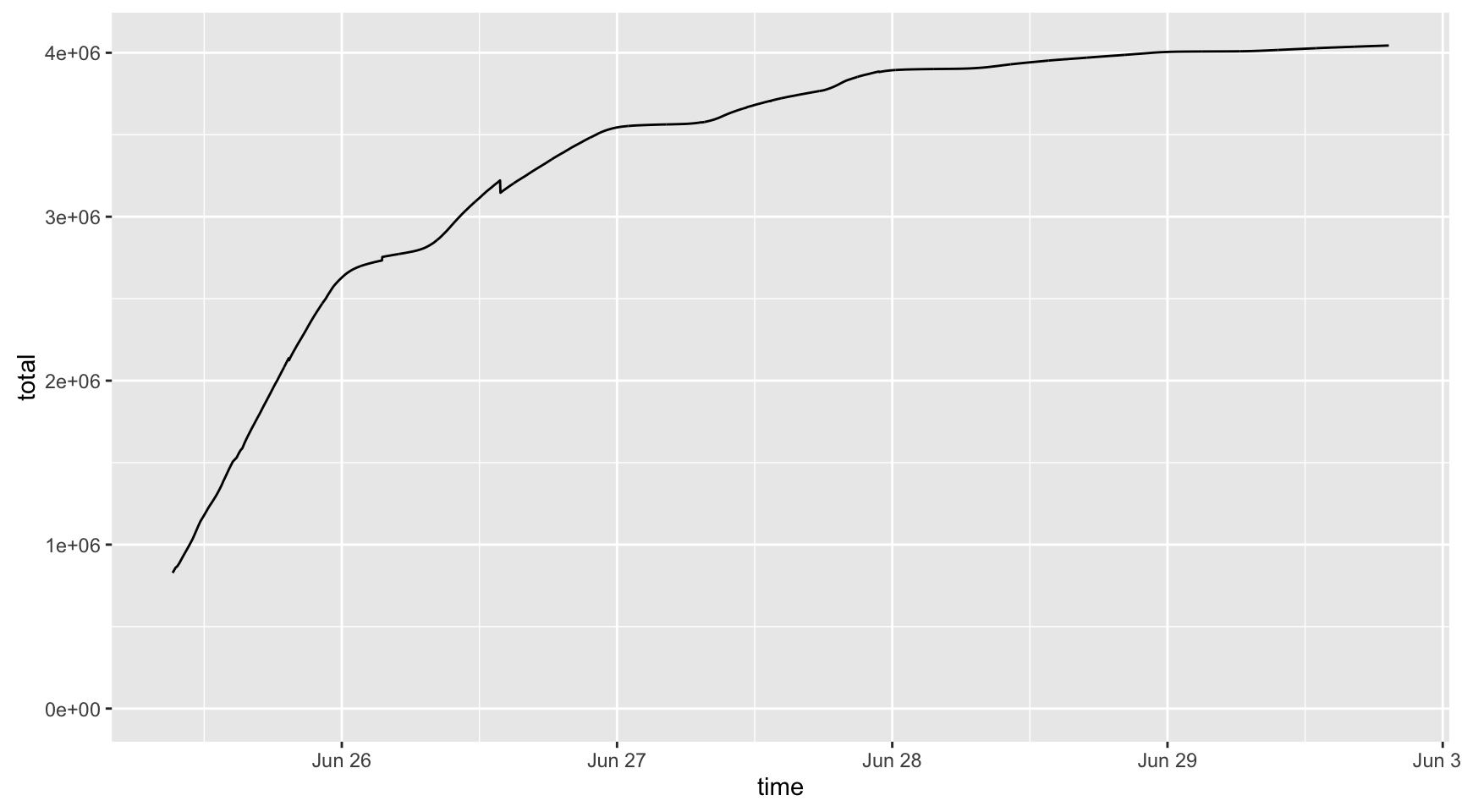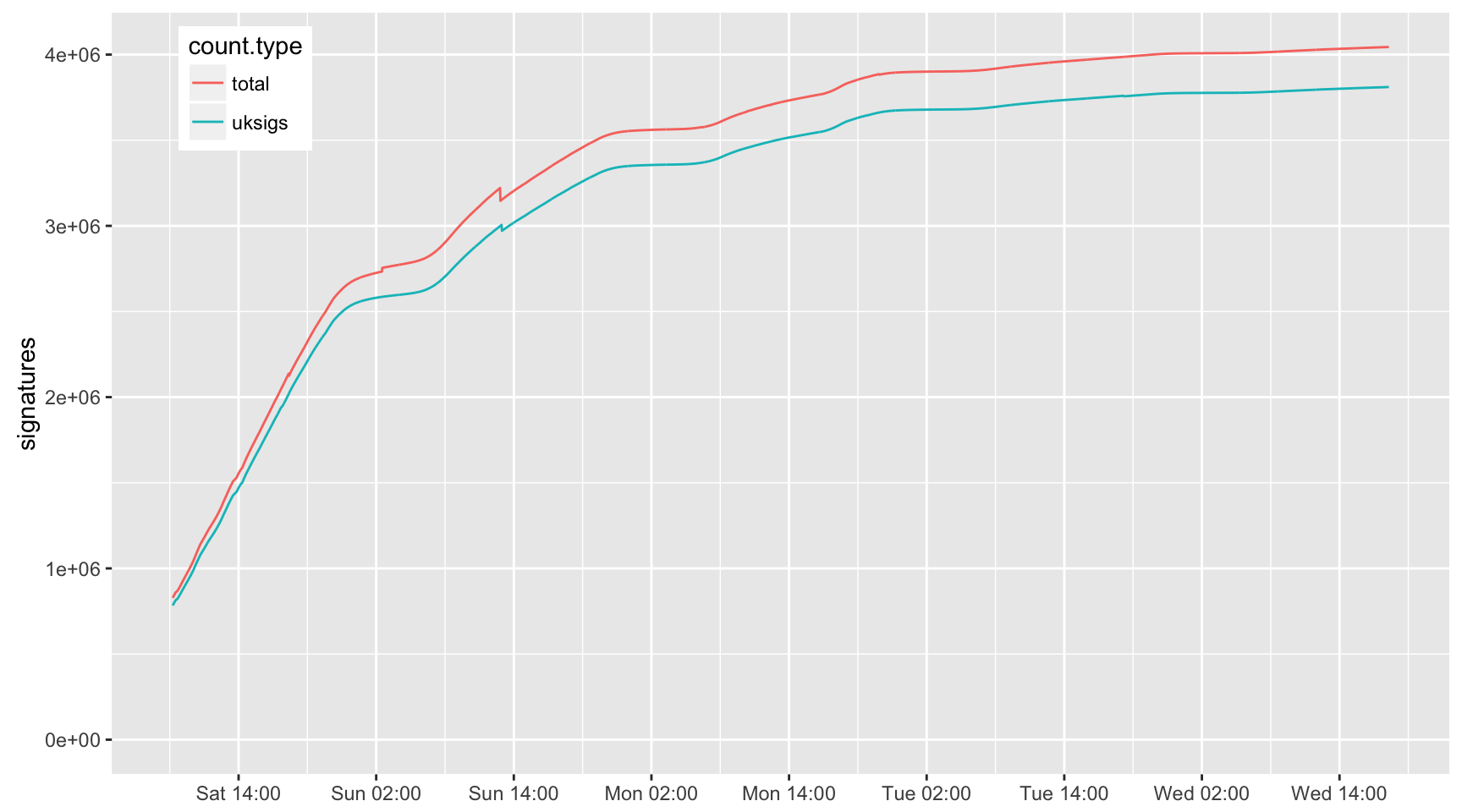# Introduction

I was curious about the EU Referendum Rules triggering a 2nd EU Referendum petition visible at https://petition.parliament.uk/petitions/131215. I started downloading the petition data every 10 mins from 10 am on Sat 25th and later upped the rate to every 2 mins. There is simple analysis presented below and you will be able to do more if you want using the data (see https://github.com/jefferis/EURef2Petition)

## Summary

1. About 95% of signatories are UK residents
2. The petition was receiving about 2000 UK signatures/min at its peak
3. Support is highest in Green (15.9%, n=1) and Lib Dem (6.1%, n=8) constituencies but there is then not much of a step down to Con (5.6%) or Lab (5.0%)
4. SNP constituencies (3.1%) are signing at approx half the rate that you might expect giving the strong remain vote in Scotland.
5. At a regional level, Scotland (3.2%) and Northern Ireland (3.4%) have signature rates less than half of the South East (6.6%) or London (9.0%)
6. There is a weak but still very significant negative correlation between the proportion of older voters in a constituency and the number of signatures.
7. There is a very strong positive correlation (R^2>0.8) between constituency level referendum results and the rate of signing the petition.
8. In this model, rates for Wales and especially Scotland were lower
9. I found evidence for about 30,000 dubious signatures using UK post-codes in 2 constituencies on Sun am. petition.parliament.uk removed these within hours (without any input from me).
10. About 3340 additional fake signatures were added on Mon later afternoon/evening with a postcode in the Bracknell constituency.
11. There were a similar number of irregularities in non-UK signatures (at a higher proportional rate since the number of non-UK signatures is only 5% of the total). I did not analyse these further since they are not relevant to the petition process.

``````# summary data frame
# list of all raw data

We can get plot the total signatures and get a quick estimate of the number of signatures per minute since I started collecting data.

``````library(ggplot2)

qplot(time, total, data=sdf, ylim=c(0,NA), geom='line')````````````mylm=lm(total~time, data=sdf)
summary(mylm)``````
``````##
## Call:
## lm(formula = total ~ time, data = sdf)
##
## Residuals:
##      Min       1Q   Median       3Q      Max
## -1685282  -136115    63757   234621   329373
##
## Coefficients:
##               Estimate Std. Error t value Pr(>|t|)
## (Intercept) -7.394e+09  7.460e+07  -99.12   <2e-16 ***
## time         5.042e+00  5.085e-02   99.17   <2e-16 ***
## ---
## Signif. codes:  0 '***' 0.001 '**' 0.01 '*' 0.05 '.' 0.1 ' ' 1
##
## Residual standard error: 289000 on 2988 degrees of freedom
## Multiple R-squared:  0.767,  Adjusted R-squared:  0.7669
## F-statistic:  9834 on 1 and 2988 DF,  p-value: < 2.2e-16``````

We can repeat the plot but only with UK signatures (although British citizens abrorad have the right to sign there is more data available for UK residents)

``````# the same but UK signatures only by using consituency table
sdf\$uksigs=sapply(pet_data, function(x) sum(x\$data\$attributes\$signatures_by_constituency\$signature_count))

# ggplot needs data in a *tall* rather than wide format
library(tidyr)
sdftall=gather(sdf[-1],count.type, n, -time)

qplot(time, n, col=count.type, data=sdftall, geom='line',
ylim=c(0,NA), ylab='signatures', xlab=NULL) +
scale_x_datetime(date_labels="%a %H:%M", date_breaks="12 hours") +
theme(legend.position = c(0.1, .9))``````Note that the 3810617 UK signatures make up 94.2213581% of the total.

You can see a couple of obvious dislocations. The up-tick of about 10,000 non-UK signatures shortly after 3am Sunday obviously looks dubious and is characterised in further detail below.

## Signatures per min

We can take a look at how the number of signatures per minute has evolved:

``````with(sdf,
qplot(time[-1],
diff(total)/(as.integer(diff(time))/60),
ylim=c(0,NA),
ylab="Signatures /min",
xlab='Time') +
scale_x_datetime(date_labels="%a %H:%M", date_breaks="12 hours")
+stat_smooth(method = 'loess', span=.03)
)``````
``## Warning: Removed 6 rows containing non-finite values (stat_smooth).``
``## Warning: Removed 6 rows containing missing values (geom_point).``